How to Use SPSS to run a linear regression and a One-Way ANOVA.

CASE STUDY: REGRESSION AND ANOVA

• ADDSCC: This variable corresponds to a continuous variable, with the ratio level of measurement. We obtain the following descriptive statistics from SPSS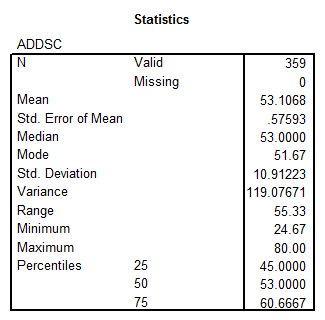• GPA: This variable corresponds to a continuous variable, with the ratio level of measurement. We obtain the following descriptive statistics from SPSS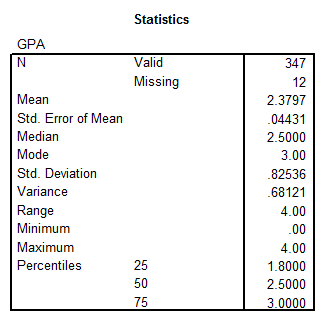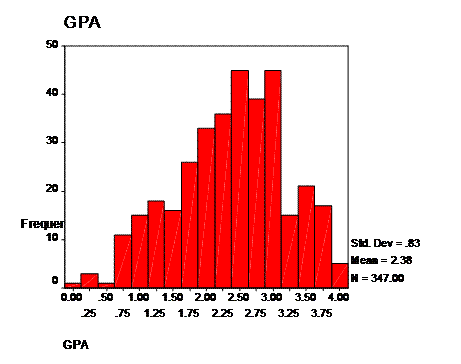• DROPOUT: This variable corresponds to a discrete variable, with the nominal level of measurement. The descriptive statistics are shown below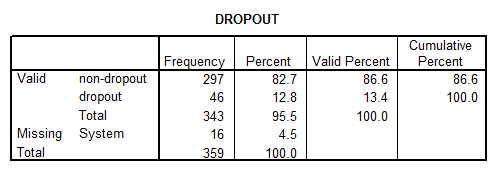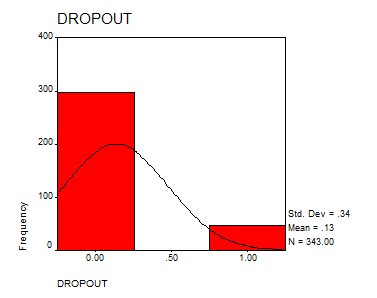• ENGL: This is a discrete variable with the nominal level of measurement. The descriptive statistics we obtain with SPPS are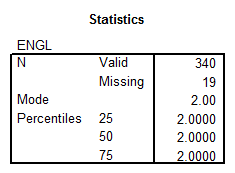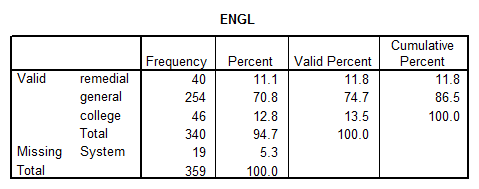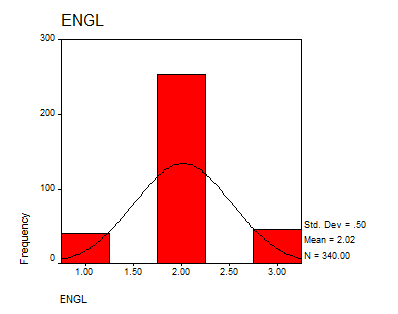• GENDER: This is a discrete variable with the nominal level of measurement. The descriptive statistics are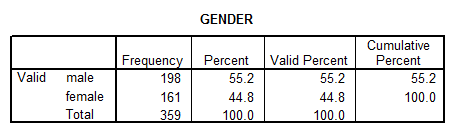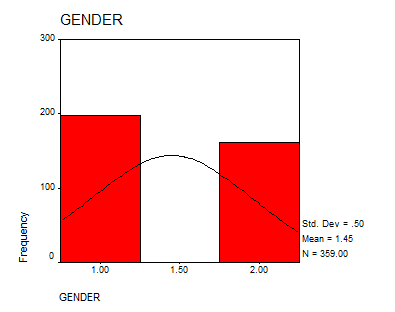• REPEAT: This is a discrete variable with the nominal level of measurement. The basic descriptive statistics are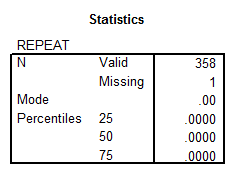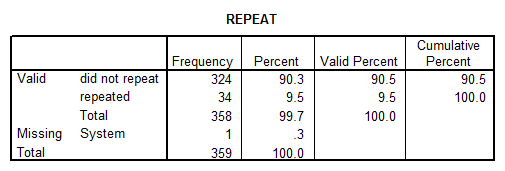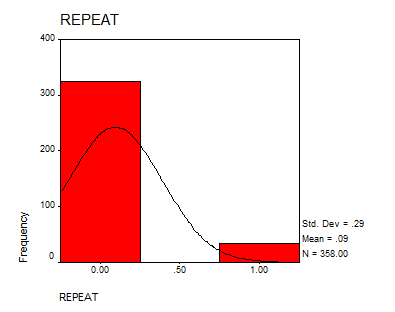• IQ: This variable corresponds to a continuous variable with the ratio level of measurement. The descriptive statistics we find with SPSS are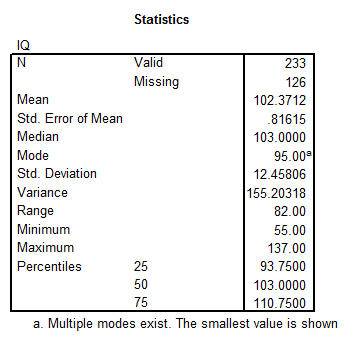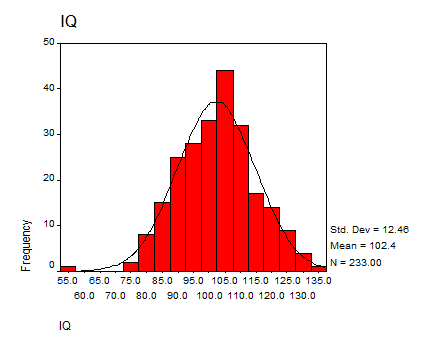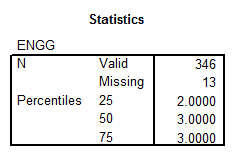• SOCPROB: This variable corresponds to a discrete variable, with the ordinal level of measurement. The descriptive statistics are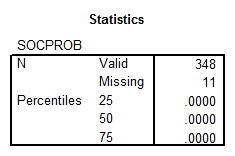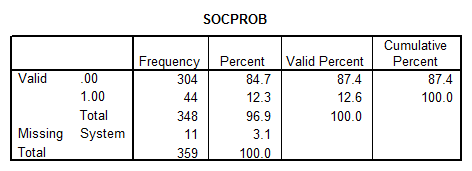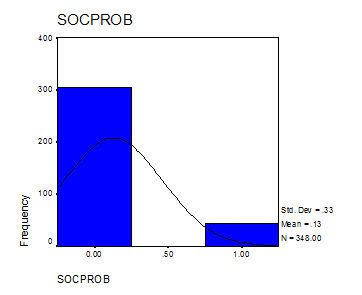3C. DIFFERENCE AMONG GROUPS

\begin{align} & {{H}_{0}}\text{: }{{\mu }_{{{G}_{1}}}}={{\mu }_{{{G}_{2}}}}\text{=}{{\mu }_{{{G}_{3}}}}\text{ } \\ & {{H}_{A}}\text{: the means are not equal}\, \\ \end{align}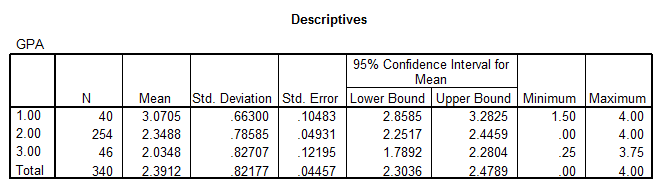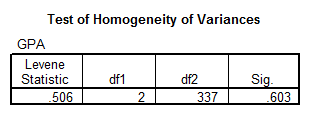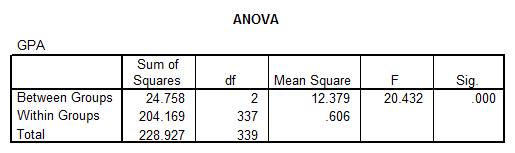• Now that we know that there is a difference between the means, we need to perform a Post-Hoc analysis, which is shown below:
• The mean of the group 1 is greater than the other group means.
• The mean of group 2 is higher than the mean of group 3.

3D. INDEPENDENCE OF CATEGORICAL VARIABLES

\begin{align} & {{H}_{0}}\text{: ''Gender''}\text{ and ''Repeat'' are independent} \\ & {{H}_{A}}:\,\text{ ''Gender''}\text{ and ''Repeat'' are NOT independent} \\ \end{align}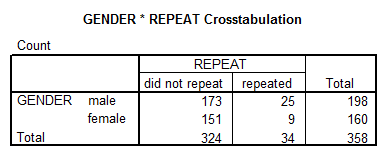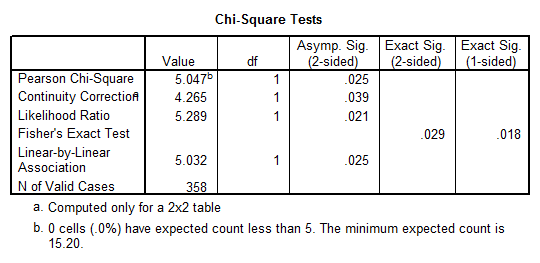• The Chi-Square is significant, with a p-value of $$p = 0.025$$, which implies that we have GENDER and REPEAT are dependent, at the $$\alpha =0.05$$ significance level.

3E. EXTRA RESEARCH QUESTION

Using SPSS: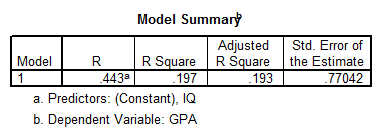$r=0.443$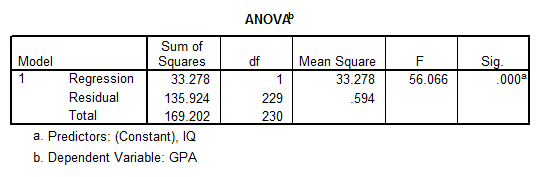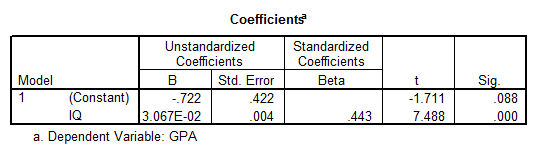$\hat{y}=-7.22+0.03067x$